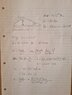# Astrophysics Redshift Question

stuartgriffin
Homework Statement:
A galaxy cluster of mass 3.0 × 1013M⊙ with known distance of 20 Mpc is observed in the centre of a bright ring, which is presumably a lensed galaxy. The angular radius of the ring from the cluster is 1.13 arcmin. The ring is observed to have a redshift of 0.0075. Assuming a value of 70kms−1Mpc−1 for the Hubble constant, determine whether the ring’s redshift distance is consistent at an accuracy of ~5% to the distance expected for the lensed galaxy to actually be located directly behind the cluster.
Relevant Equations:
a=4GM/bc^2
V=H0D
I have attempted to link the equations, but I don't really understand how the data given fits. Does the angular radius get plugged in as the deflection angle?

Staff Emeritus
Homework Helper
Gold Member
What is the distance to the lensed galaxy? How can this help you get the deflection angle?

•stuartgriffin
stuartgriffin
What is the distance to the lensed galaxy? How can this help you get the deflection angle?

Ahhh, thank you, so I calculate the distance using the redshift and hubbles constant? Am I on the right lines with the equations I am using?

stuartgriffin
What is the distance to the lensed galaxy? How can this help you get the deflection angle?
Does this look like I'm headed on the right track?

#### Attachments

•16170999329211369232338957203962.jpg
48.4 KB · Views: 83